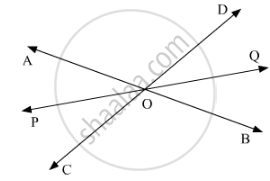# If Two Straight Lines Intersect Each Other, Prove that the Ray Opposite to the Bisector of One of the Angles Thus Formed Bisects the Vertically Opposite Angle. - Mathematics

If two straight lines intersect each other, prove that the ray opposite to the bisector of one of the angles thus formed bisects the vertically opposite angle.

#### Solution

Let AB and CD intersect at a point OAlso, let us draw the bisector OP of ∠AOC.

Therefore,

∠AOP = ∠POC       (1)

Also, let’s extend OP to Q.

We need to show that, OQ bisects ∠BOD.

Let us assume that OQ bisects∠BOD, now we shall prove that POQ is a line.

We know that,

∠AOCand ∠DOBare vertically opposite angles. Therefore, these must be equal, that is:

∠AOC = ∠DOB       (2)

∠AOPand ∠BOQ are vertically opposite angles. Therefore,

∠AOP = ∠BOQ

Similarly,

∠POC = ∠DOQ

We know that:

∠AOP +∠AOD+∠DOQ+∠POC+∠BOC+∠BOQ = 360°

2∠AOP+∠AOD+2∠DOQ+∠BOC =360°

2∠AOP + 2∠AOD+ 2∠DOQ = 360°

2(∠AOP+∠AOD+ ∠DOQ) = 360°

∠AOP+∠AOD+ ∠DOQ = (360°)/2

∠AOP+∠AOD+ ∠DOQ = 180°

Thus, POQ is a straight line.

Hence our assumption is correct. That is,

We can say that if the two straight lines intersect each other, then the ray opposite to the bisector of one of the angles thus formed bisects the vertically opposite angles.

Concept: Introduction to Lines and Angles
Is there an error in this question or solution?
Chapter 10: Lines and Angles - Exercise 10.3 [Page 23]

#### APPEARS IN

RD Sharma Mathematics for Class 9
Chapter 10 Lines and Angles
Exercise 10.3 | Q 13 | Page 23
Share